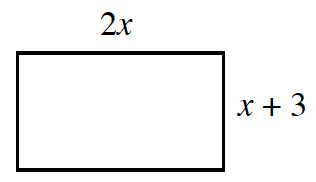Home > GB8I > Chapter cc43 > Lesson cc43.1.3 > Problem3-33

3-33.Examine the rectangle at right. Homework Help ✎

1. Write an expression for the perimeter in terms of x.

The perimeter is all of the sides added together, or 2l + 2w.

2. Assuming the perimeter is 78 cm, determine the dimensions of the rectangle. Show all of your work.

6x + 6 = 78
6x = 72
Solve for x and then substitute for the x in the expressions for the length and the width.

3. Verify that the area of this rectangle is 360 sq. cm. Explain how you know this.

• In part (b), You found x. Now find the length and width.
The area is equal to the length times the width.